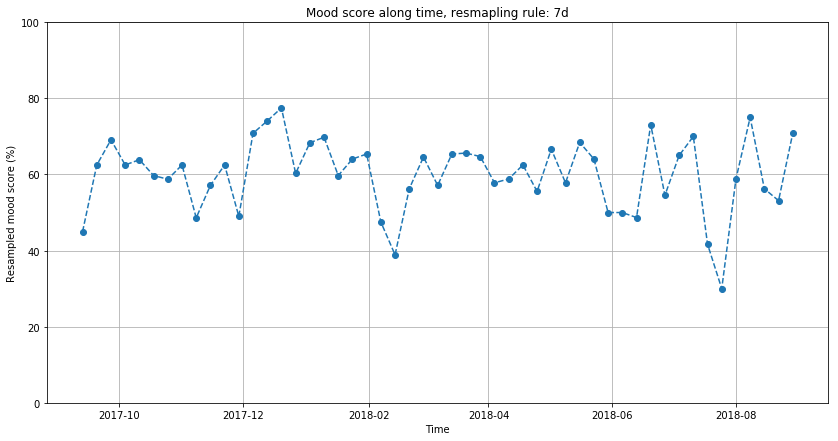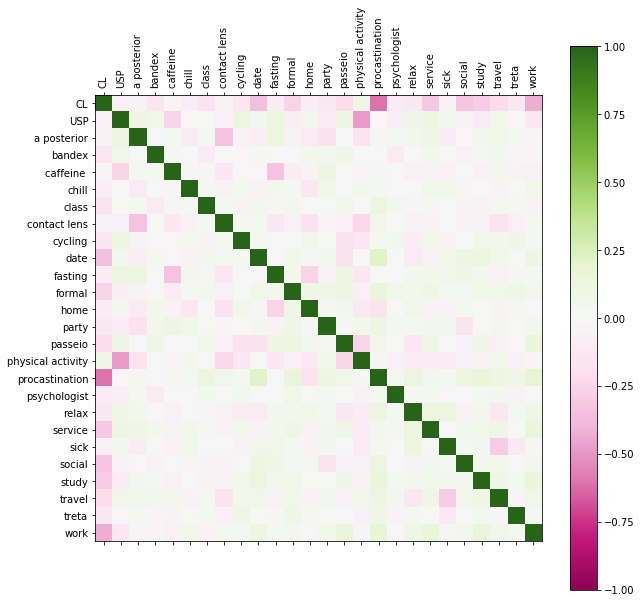# Details for `Daylio Analysis.ipynb`

#### Description

Notebook for reading and processing an Daylio exported csv to a more friendly dataset and doing an quick visualization of it.

In this notebook, I perform an resampled visualization of the mood score and an visualization of the correlation matrix between the different activities when doing an multilinear fit on the data.

But the best visualization is the results of the multilinear fit on the activities - it summarizes the relations between the activities and the mood

daylio daylio

#### Comments

Right now this notebook isn't tied in into the Open Humans API but rather relies on the presence of a `daylio_export.csv` file in the same folder as the Notebook. Would be cool to get this tied in with the API though!

Please log in to comment.

# Notebooks para estudar os dados do Daylio¶

## Imports¶

In :
```import pandas as pd
import numpy as np
import matplotlib.pyplot as plt
import scipy.stats as st
import scipy.optimize as opt
from pandas.tools.plotting import table
import statsmodels.api as sm
import scipy.fftpack as fft
```

## Custom definitions¶

In :
```# Put here the score for each custom mood in Daylio
mood_score = {"awful": 0, "melancholic": 0, "aaa": 0,
"bad": 1, "shitty": 1, "grr": 1,
"aff":2, "zzz": 2, "meh": 2,
"good": 3, "satisfied": 3, "gogo": 3,
"rad": 4, "wonderful": 4, "hihi": 4}

# When doing visualizations and data-fitting, only consider activities with more than N points
activities_N_minimum = 19
```

## Data preparation¶

In :
```# Preparar dados do humor
raw_mood_data = pd.read_csv("daylio_export.csv", parse_dates=[[0, 1, 2, 3]], index_col="year_date_weekday_time")
raw_mood_data = raw_mood_data.replace({"mood": mood_score}).copy()
raw_mood_data = raw_mood_data.replace(np.nan, "")
mood_data = raw_mood_data.copy()

# Normalize mood score to an domain between 0 and 100
mood_data.mood *= 100
mood_data.mood /= 4
```
In :
```# Separate the activities on each row

activities_string = " | ".join(mood_data.activities.values)
activities_string = activities_string.split(" | ")
unique_activities = np.unique(activities_string)
unique_activities = unique_activities[unique_activities != ""]
mood_data.activities = mood_data.activities.str.split(" \| ")
```
In :
```# Generate new columns with the activities
for activity in unique_activities:
contains_activity = mood_data.activities.apply(lambda x: activity in x)
mood_data[activity] = contains_activity
```

## Visualization¶

In :
```resample_rule = "7d"

daily_mood = mood_data.resample(resample_rule).mean().mood
```
In :
```plt.figure(figsize=(14, 7))
plt.grid()
plt.plot(daily_mood, "--o")
#plt.plot(mood_data[mood_data.sick == True].mood, 'o')
plt.ylim((0, 100))
plt.title("Mood score along time, resmapling rule: {}".format(resample_rule))
plt.ylabel("Resampled mood score (%)")
plt.xlabel("Time")
plt.show()
```In :
```# Get list of activities which has more than activities_N_minimum points (user-defined)

unique_activities_2 = []
for activity in unique_activities:
if mood_data[activity].sum() > activities_N_minimum:
unique_activities_2.append(activity)
```
In :
```# Do an multilinear fitting on the data

N_samples = len(mood_data)
N_features = len(unique_activities_2)
features = []

X = np.zeros((N_samples, N_features))
Y = np.array(mood_data.mood.values)
for i in range(N_features):
feature = unique_activities_2[i]
features.append(feature)
X[:, i] = mood_data[feature].astype(int)

X = sm.add_constant(X)
est = sm.OLS(Y, X).fit()

summary = est.summary()
cov_mtr = est.cov_params() # covariance matrix
diag = np.diag(np.diag(cov_mtr))
D = np.linalg.inv(np.sqrt(diag))
r = np.matmul(D, np.matmul(cov_mtr, D)) # correlation matrix
```
In :
```# Plot the correlation matrix for the multilinear fit

fig = plt.figure(figsize=(10, 10))
ax = fig.add_subplot(111)
mtr = ax.matshow(r, vmin=-1, vmax=1, cmap="PiYG")
plt.colorbar(mtr)
ax.set_xticks(np.arange(r.shape))
ax.set_xticklabels(["CL"] + unique_activities_2, rotation="vertical")
ax.set_yticks(np.arange(r.shape))
ax.set_yticklabels(["CL"] + unique_activities_2, rotation="horizontal")
plt.show()
```In :
```# Transforming the summary table into something more readable

i = 0
for activity in unique_activities_2:
summary.tables[2:][i].data = activity
i += 1
```
In :
```# Show the summary of the multilinear fit

# Rough (and technically wrong) meanings of the columns
# [coef] how much (+/-)% the activity has influence on the mood score (on average)
# [std err] the dispersion of the [coef]
# [t] measure of the reliability for the [coef] estimation.
# |t|=2 means about 95% chance of being right and |t|>2 means about 99% chance of being ok. |t|=1 is about 68%

summary
```
Out:
Dep. Variable: R-squared: y 0.291 OLS 0.269 Least Squares 13.22 Tue, 04 Sep 2018 4.00e-45 16:08:12 -3571.3 833 7195. 807 7317. 25 nonrobust
coef std err t P>|t| [0.025 0.975] 54.5210 1.586 34.373 0.000 51.407 57.634 5.1119 2.533 2.018 0.044 0.139 10.085 -2.7219 2.250 -1.210 0.227 -7.139 1.695 1.5685 2.852 0.550 0.582 -4.029 7.166 0.3053 2.114 0.144 0.885 -3.843 4.454 6.8547 3.209 2.136 0.033 0.557 13.153 -1.8198 3.432 -0.530 0.596 -8.557 4.918 -1.7243 2.110 -0.817 0.414 -5.866 2.418 0.9111 2.520 0.361 0.718 -4.036 5.858 6.4735 1.646 3.932 0.000 3.242 9.705 2.2845 2.460 0.929 0.353 -2.545 7.114 1.1135 2.548 0.437 0.662 -3.887 6.114 -3.5779 1.865 -1.918 0.055 -7.239 0.083 -2.6290 3.938 -0.668 0.505 -10.359 5.101 5.7355 1.851 3.099 0.002 2.103 9.368 0.5302 2.401 0.221 0.825 -4.183 5.243 -2.5270 1.435 -1.761 0.079 -5.343 0.289 1.1910 3.367 0.354 0.724 -5.418 7.800 16.0155 2.854 5.612 0.000 10.414 21.617 3.7127 1.827 2.032 0.042 0.126 7.299 -10.0804 3.203 -3.147 0.002 -16.368 -3.793 11.8796 1.422 8.354 0.000 9.088 14.671 -0.5976 2.241 -0.267 0.790 -4.997 3.802 -4.5606 2.446 -1.864 0.063 -9.362 0.241 -22.7499 2.793 -8.146 0.000 -28.232 -17.268 5.1111 1.599 3.197 0.001 1.973 8.249
 Omnibus: Durbin-Watson: 24.242 1.463 0 25.602 -0.412 2.76e-06 3.242 8.87
In [ ]:
```
```
In [ ]:
```
```